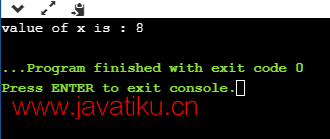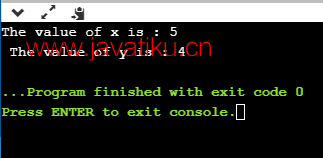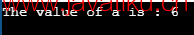# C语言教程-C语言中的解引用指针## 为什么使用解引用指针？

• 它可以用于访问或操作指针所指向的内存位置中存储的数据。
• 对解引用指针应用的任何操作都将直接影响它所指向的变量的值。

• 首先，我们声明一个整型变量，指针将指向该变量。
``int x = 9;``
• 然后，我们声明一个整型指针变量。
``int *ptr;``
• 在整型指针变量的声明之后，我们将'x'变量的地址存储到指针变量'ptr'中。
``ptr = &x;``
• 我们可以通过对指针'ptr'进行解引用来更改'x'变量的值，如下所示：
``*ptr = 8;``

``````#include <stdio.h>

int main() {
int x = 9;
int *ptr;
ptr = &x;
*ptr = 8;
printf("x的值为：%d", x);
return 0;
}````````````#include <stdio.h>

int main() {
int x = 4;
int y;
int *ptr;
ptr = &x;
y = *ptr;
*ptr = 5;
printf("x的值为：%d\n", x);
printf("y的值为：%d\n", y);
return 0;
}``````

• 我们声明了两个变量'x'和'y'，其中'x'存储了值'4'。
• 我们声明了一个指针变量'ptr'。
• 在声明指针变量后，我们使用指针'ptr'将'x'变量的地址赋值给'y'，即y = *ptr，而不是使用'x'变量。``````#include <stdio.h>

int main() {
int a = 90;
int *ptr1, *ptr2;
ptr1 = &a;
ptr2 = &a;
*ptr1 = 7;
*ptr2 = 6;
printf("a的值为：%d\n", a);
return 0;
}``````

• 首先，我们声明一个变量'a'。
• 然后，我们声明两个指针变量'ptr1'和'ptr2'。
• 两个指针都包含'a'变量的地址。
• 我们将'ptr1'赋值为'7'，将'ptr2'赋值为'6'。最终，'a'的值将变为'6'。